ConceptDraw
DIAGRAM 14

# Diagramming Software for Design UML Object Diagrams

## UML Object Diagrams Designing

UML Object Diagram shows the structure of a modeled system at a specific time. UML Object Diagram focuses on some particular set of object instances and attributes, and the links between the instances. A correlated set of object diagrams provides insight into how an arbitrary view of a system is expected to evolve over time.

ConceptDraw helps you to start designing UML Object Diagrams with examples and templates.

You can use the appropriate stencils of UML notation for drawing your own UML Object Diagram.

## UML Object Diagram Library

ConceptDraw Rapid UML solution delivers libraries contain pre-designed objects fit UML notation, and ready to draw professional UML Object Diagram.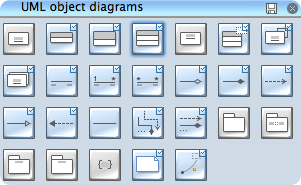Example 1. UML Object Diagram library

## UML Object Diagram Design Elements

Design elements sample shows all library objects at one page for quick review.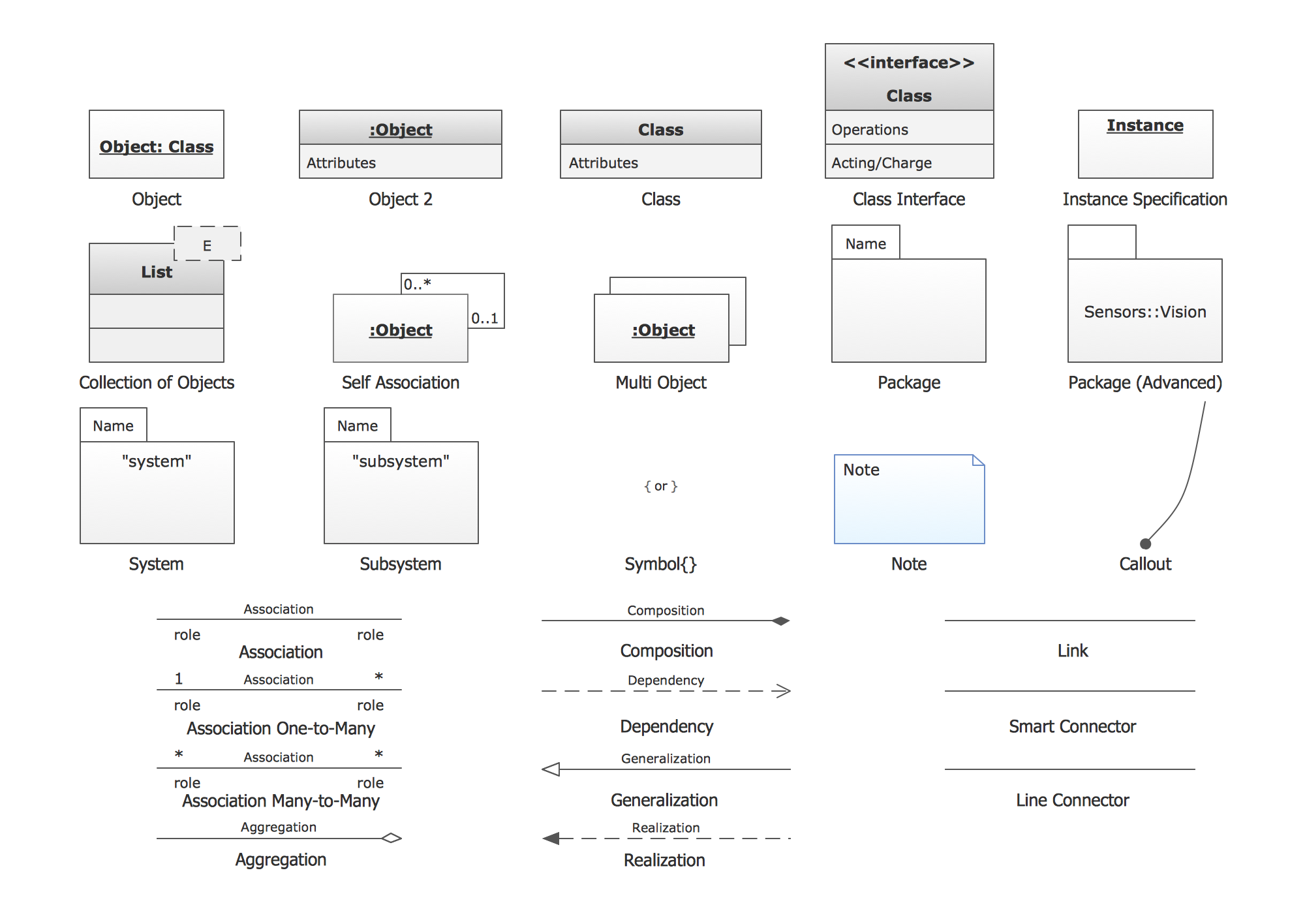Example 2. UML Object Diagram design elements

## Design UML Object Diagram in ConceptDraw DIAGRAM software

ConceptDraw DIAGRAM workspace is clear and simple. All tools you need for drawing UML Object Diagram are on hand.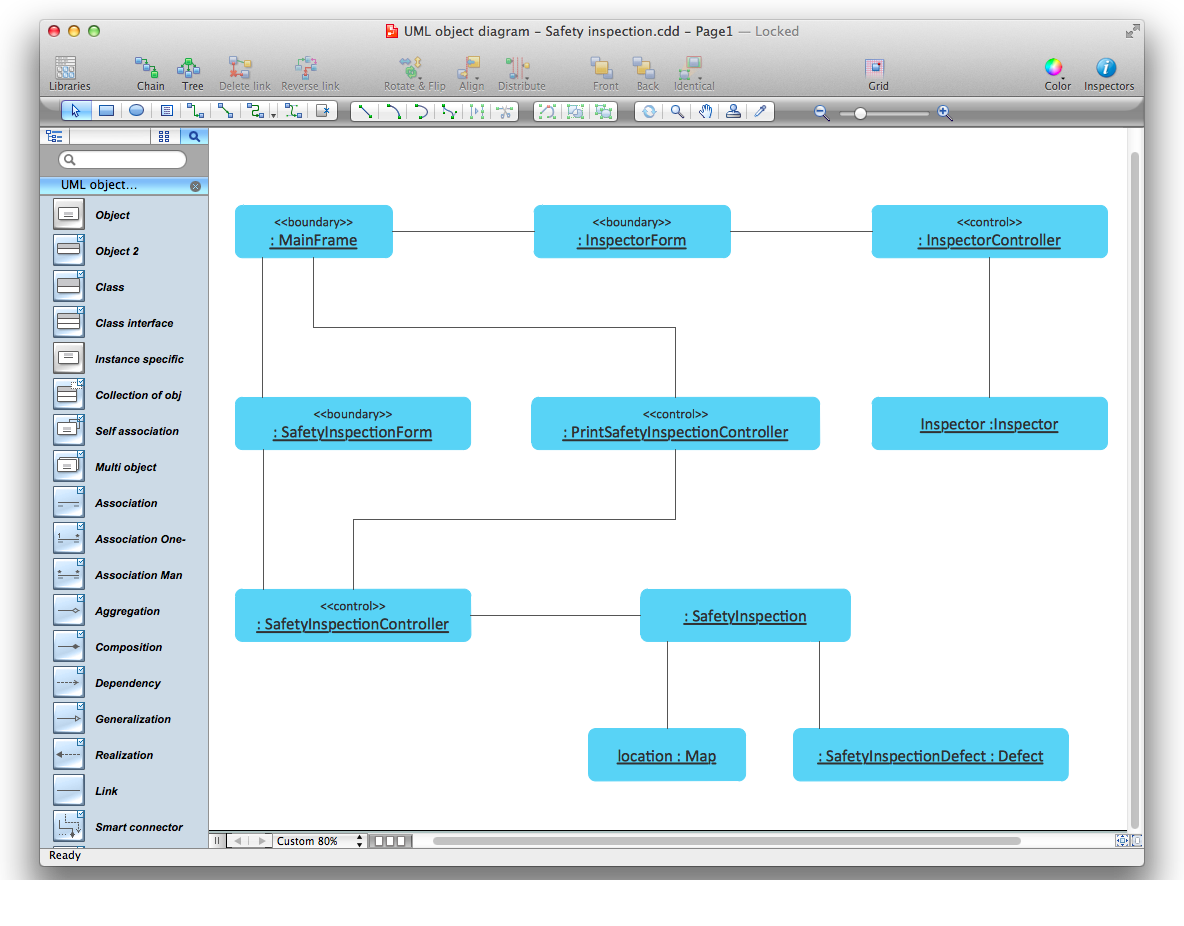Example 3. UML Object Diagram for Mac OS X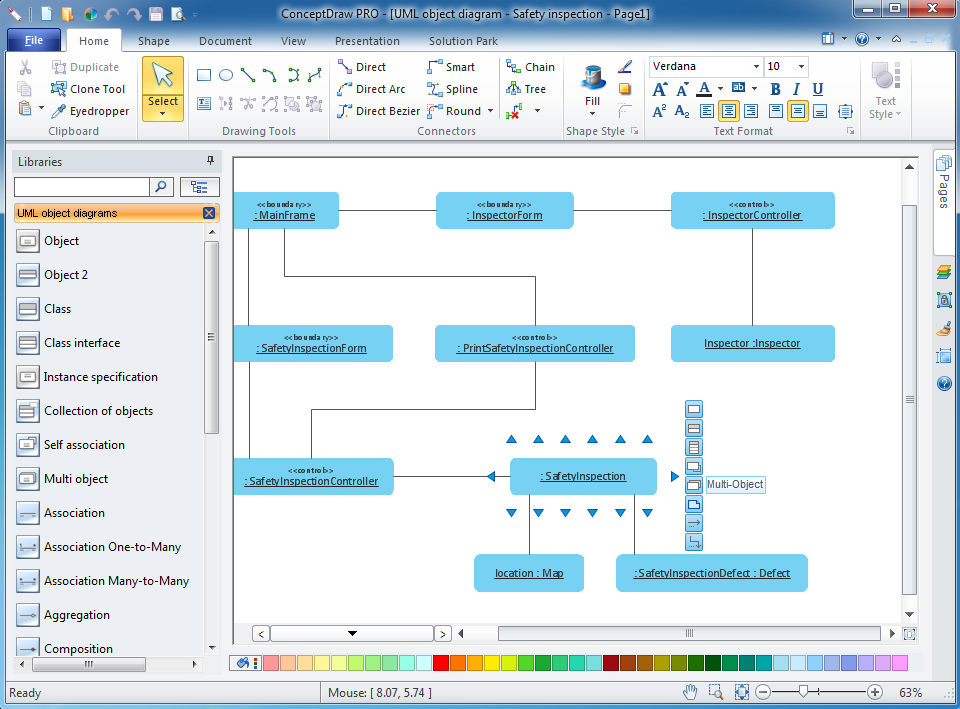Example 4. UML Object Diagram for Windows

## Examples of UML Object Diagram

This is an example of UML Object Diagram for an Safety inspection process.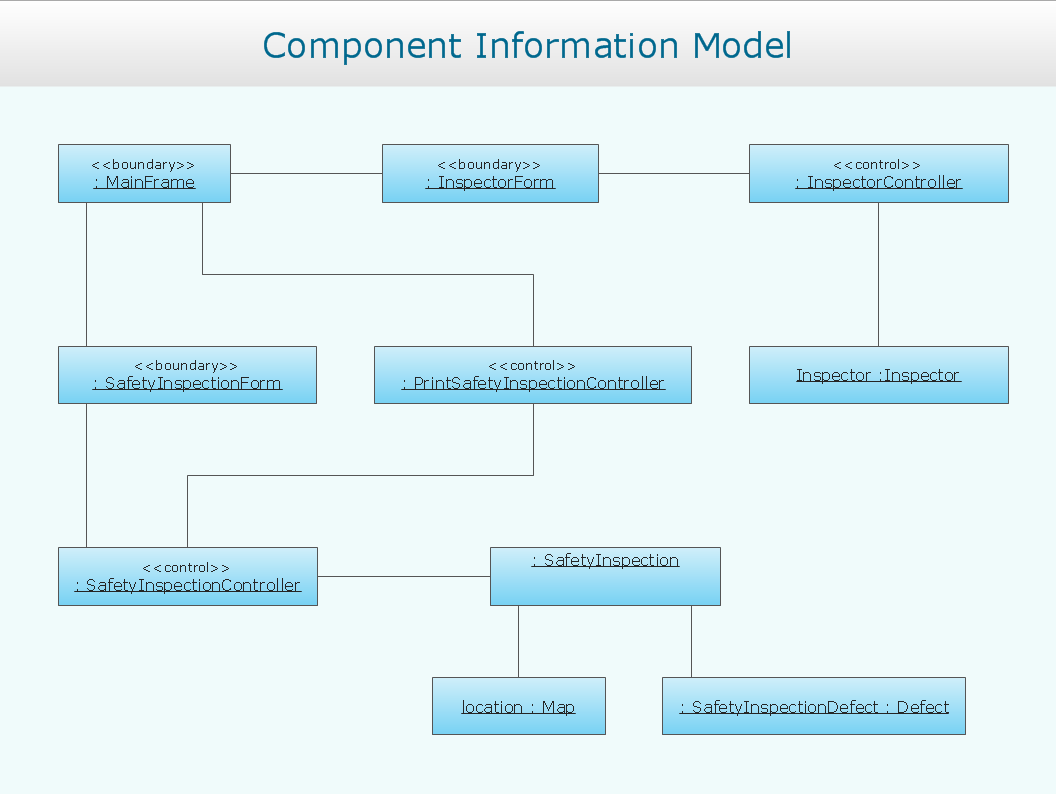Example 5. UML Object Diagram - Safety inspection

This UML object diagram sample is created using ConceptDraw DIAGRAM diagramming and vector drawing software enhanced with Rapid UML solution from ConceptDraw Solution Park.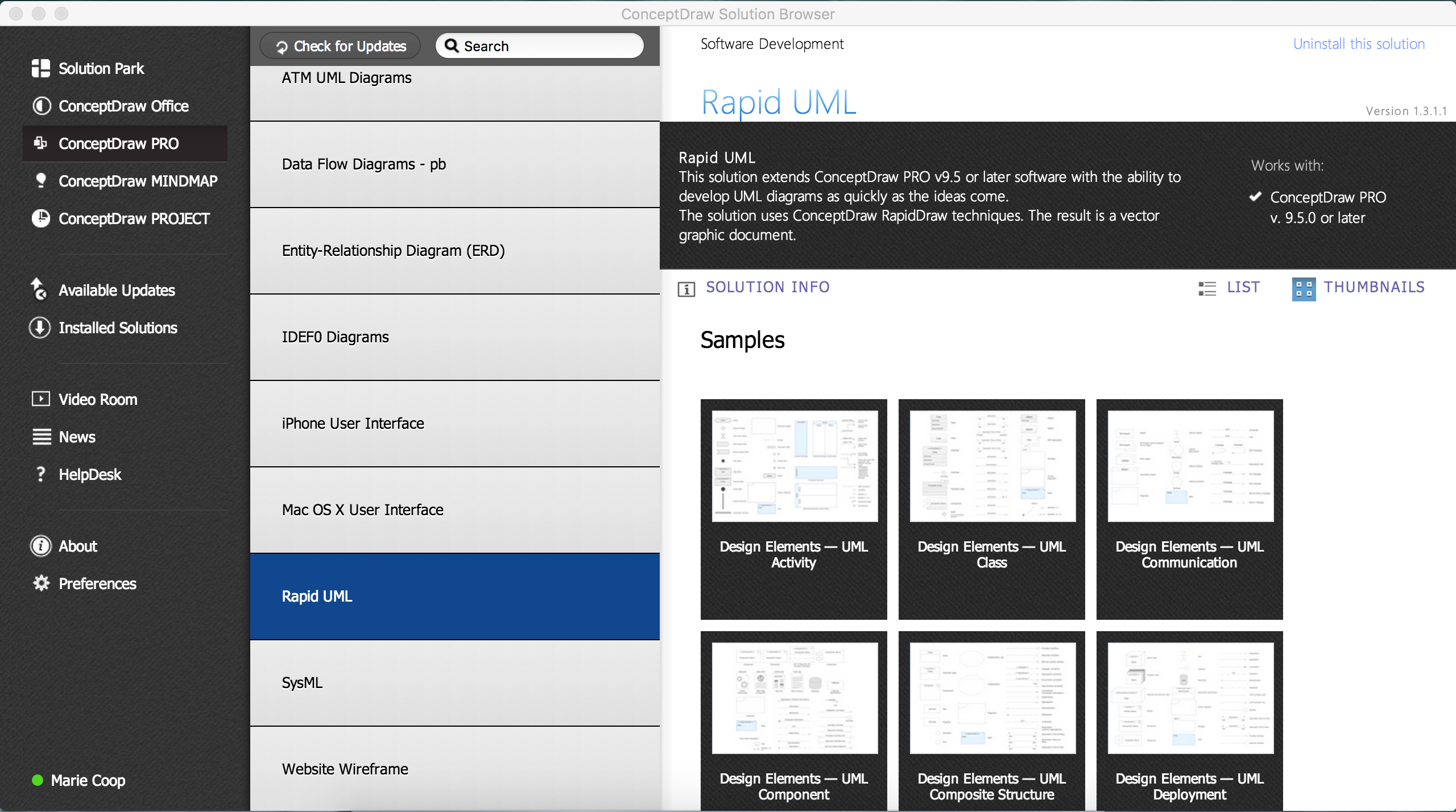Example 6. UML Object Diagram Solution

Rapid UML solution provides templates, examples and libraries of stencils for quick and easy drawing the all types of system and software engineering diagrams according to UML 2.4 notation.

Use ConceptDraw DIAGRAM with UML object diagram templates, samples and stencil library from Rapid UML solution to show the particular set of system objects, their attributes and the links between them.

### UML Notation →

There are many ways to track the system in a critical situation. To model a system behavior, uml notation is widely used. Usually, an UML diagram consists of elements such as actor and a case. This diagram represents the structure of UML notations. Unified Modeling Language (UML) is used in software engineering to depict graphically the software modeling process. UM Language uses graphic notations for developing models of object-oriented systems. These notations displays requirements, sub-systems, logical and physical elements, etc. We created this diagram using ConceptDraw DIAGRAM reinforced with Rapid UML solution. It can be helpful for students on software engineering, when learning UML.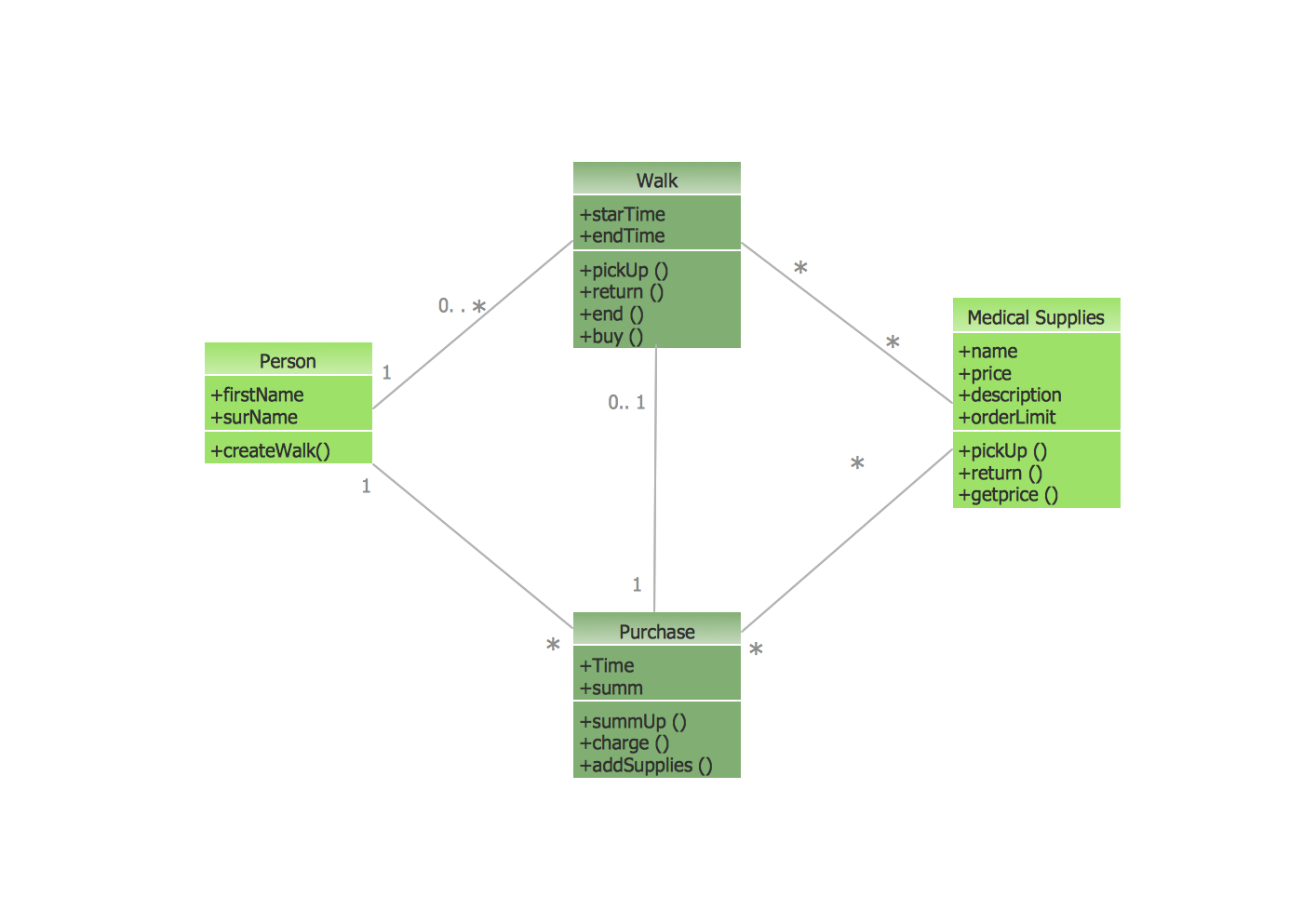Picture: UML Notation
Related Solution:

### UML Class Diagram Notation →

When it comes to system construction, a class diagram is the most widely used diagram. UML Class Diagrams is a type of static structure diagram that is used for general conceptual modeling of the systematics of the application. Such a diagram would illustrate the object-oriented view of a system. The object orientation of a system is indicated by a class diagram. It describes the structure of a system by showing the general issues,classes of a system, attributes, operations, interfaces, inheritance, and the relationships between them.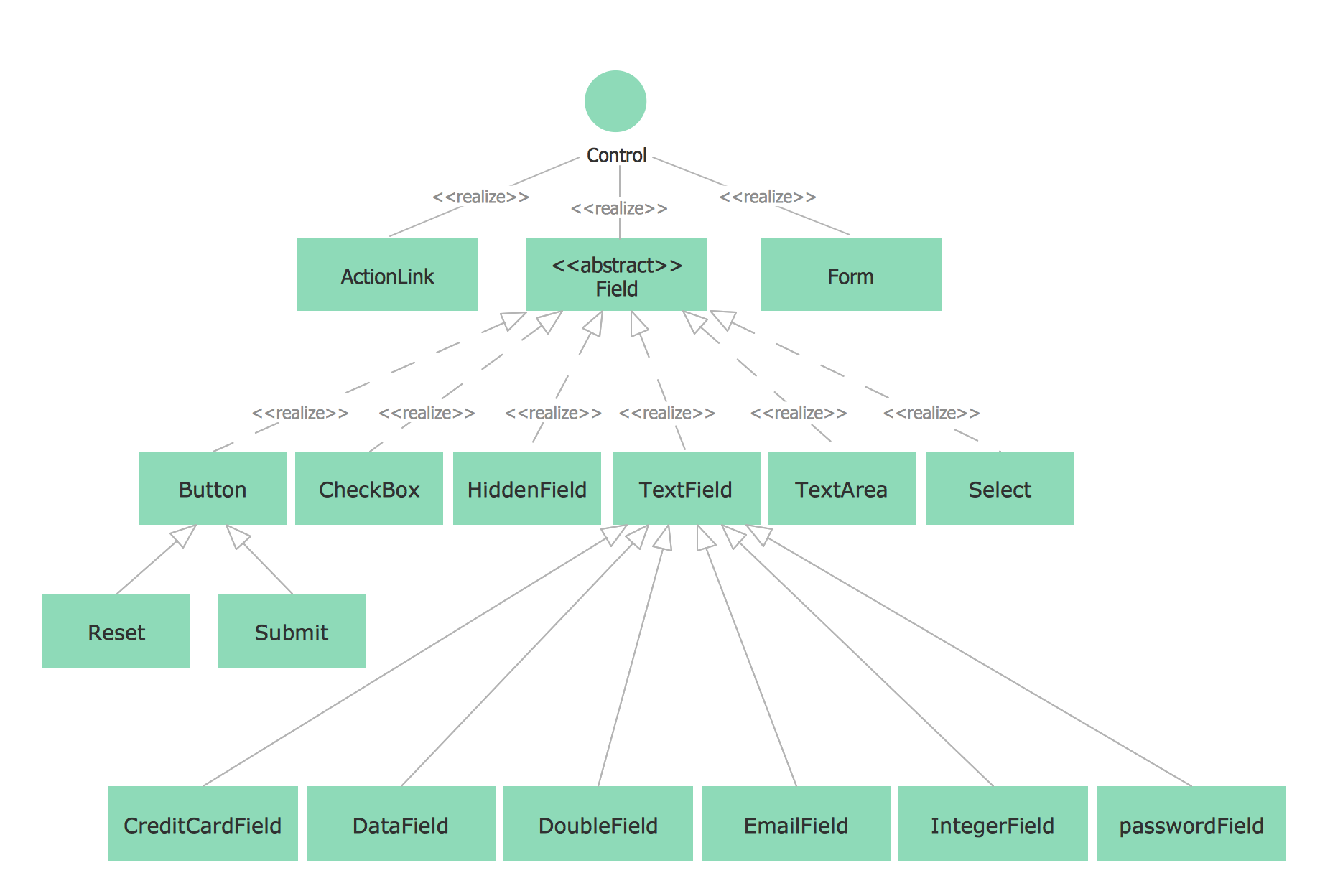Picture: UML Class Diagram Notation
Related Solution:

### UML Use Case Diagram Example. Registration System →

The use case diagram (behavior scenarios, precedents) is the initial conceptual representation of the system during its design and development. This diagram consists of actors, use cases and relationships between them. When constructing a diagram, common notation elements can also be used: notes and extension mechanisms. This sample was created in ConceptDraw DIAGRAM diagramming and vector drawing software using the UML Use Case Diagram library of the Rapid UML Solution from the Software Development area of ConceptDraw Solution Park. This sample shows the types of user’s interactions with the system and is used at the registration and working with the database system.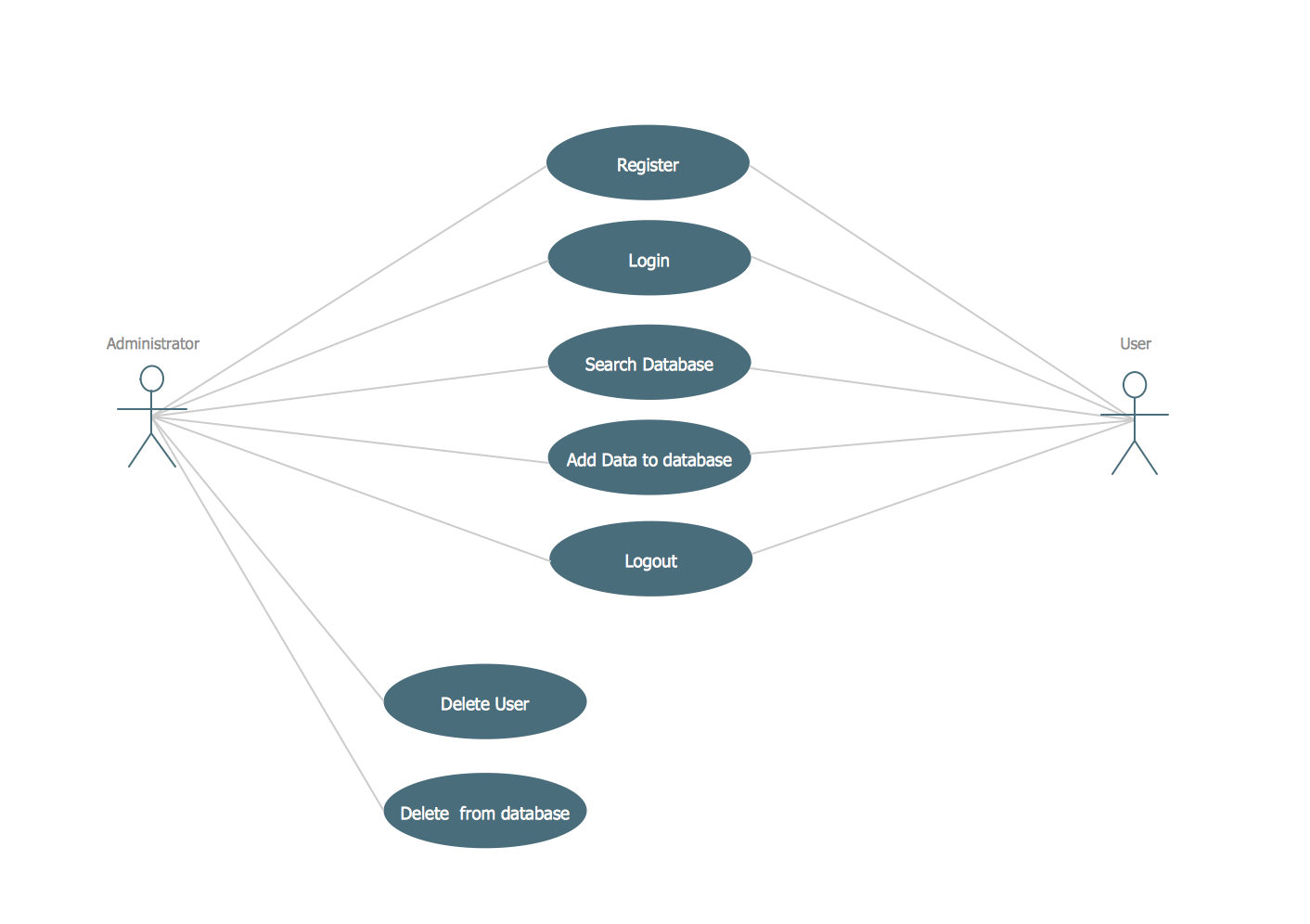Picture: UML Use Case Diagram Example. Registration System
Related Solution:

### UML Package Diagram. Design Elements →

UML Package Diagram illustrates the functionality of a software system. ConceptDraw has 393 vector stencils in the 13 libraries that helps you to start using software for designing your own UML Diagrams. You can use the appropriate stencils of UML notation from UML Package library.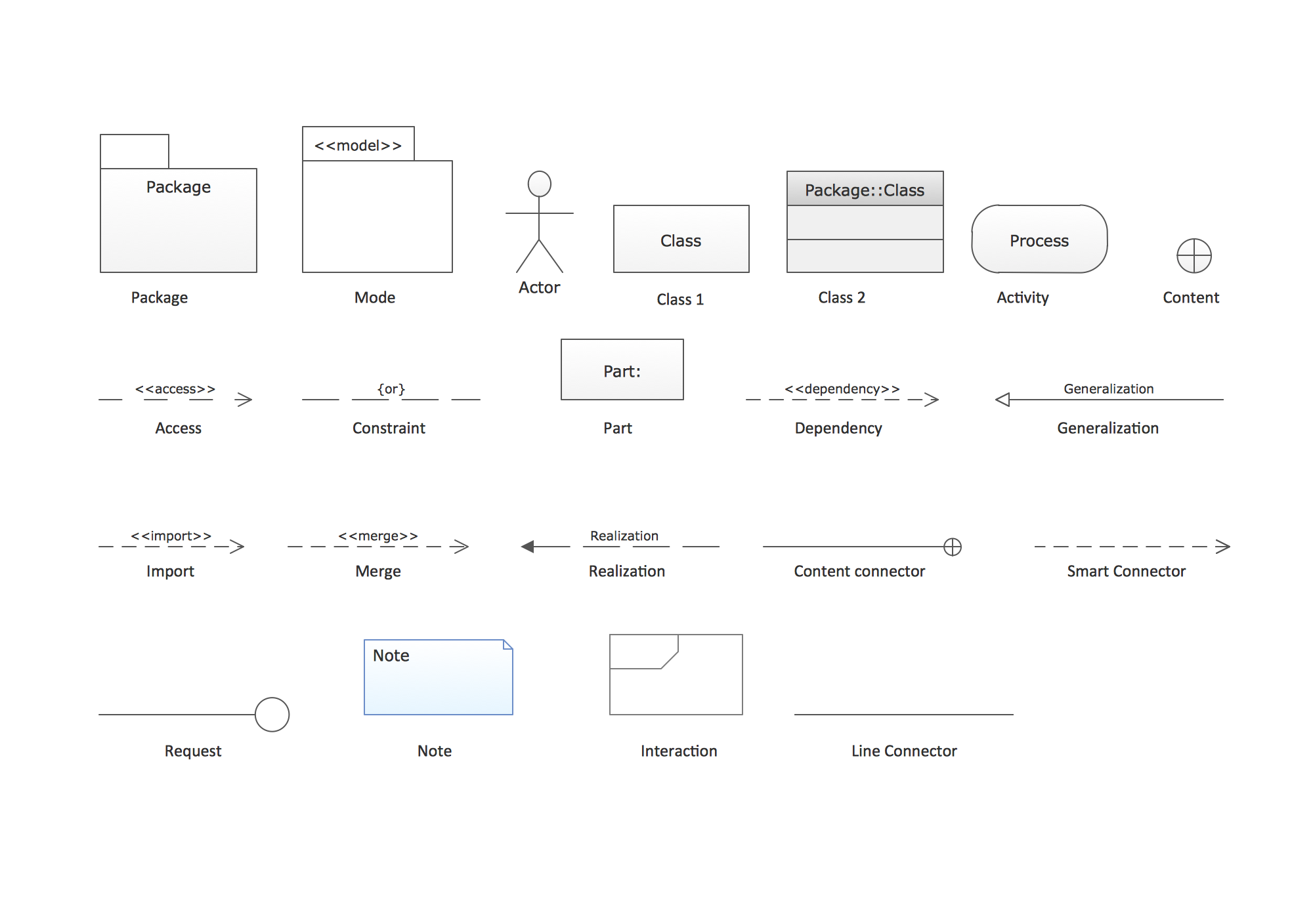Picture: UML Package Diagram. Design Elements
Related Solution:

### Data Flow Diagram →

There are many ways to define the flow of information within a system. Structured-systems analysis method often uses data flow diagrams to show data flow, data storages and data processing visualization. These diagrams are easy to develop and quite useful. Here is a dataflow diagram presenting an example of the manufacturing process flow. The oval process shapes represent a process that controls data within the current system. The process may produce data or make some action based on data. Data flows are represented by lines. They show the movement of information through the system. The direction of the data flow is depicted by the arrow. The external entities are shown by rectangles. They represent the external entities interacting with the system. The data store objects (unclosed rectangles) are used to show data bases participating in a process flow.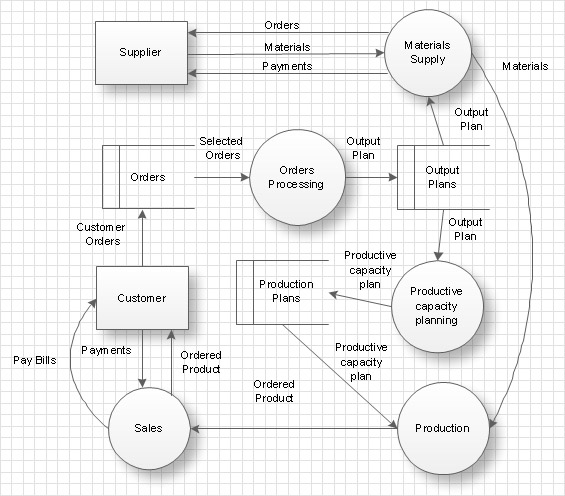Picture: Data Flow Diagram
Related Solution:

### Bank Sequence Diagram →

ConceptDraw DIAGRAM diagramming and vector drawing software enhanced with ATM UML Diagrams Solution from the Software Development Area of ConceptDraw Solution Park is a perfect tool for fast and easy creating the Bank Sequence Diagram.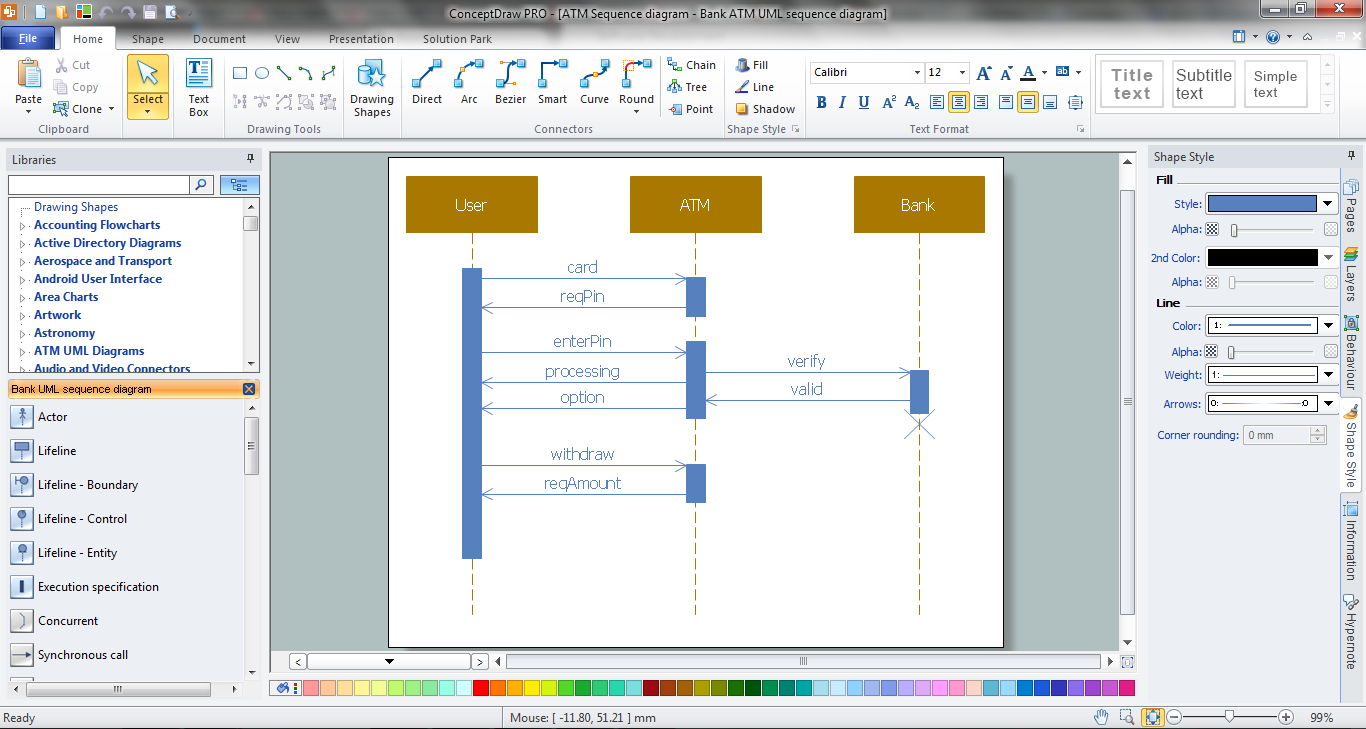Picture: Bank Sequence Diagram
Related Solution:

### UML Diagram →

While developing software, it is very important to have a visual model, because it helps to represent the logic and the architecture of an application. Experienced engineers use UML diagrams to denote relationships between classes and their instances. UML is a general language for a set of diagrams like deployment diagrams, object diagrams or use case diagrams. This diagram represents UML class diagram used for a software system development using an object-oriented method. Class diagrams are categorized as static structure diagrams that depict the physical structure of a system. Class diagram divides a software system's structure into "classes". Classes are defined by the methods and variables of objects. UML Class diagram is used to depict relationships and source code dependencies between objects.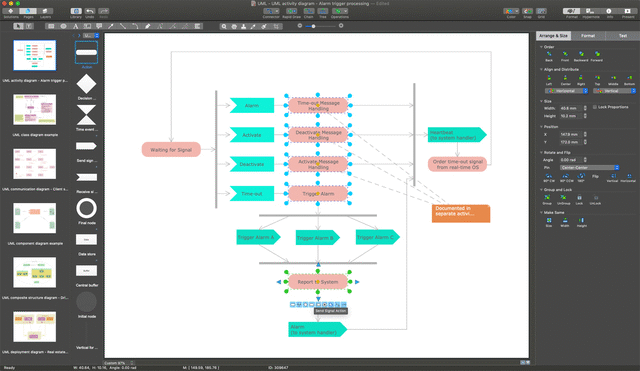Picture: UML Diagram
Related Solution:

### Object-Oriented Design →

Being involved in the process of planning some system of interacting the objects, or in other words, being engaged in the object-oriented design in order to solve some software problem as one of the approaches used in the software design, you may find the ConceptDraw DIAGRAM diagramming and drawing software a really useful tool for making the needed drawings, such as the IDEF Business Process Diagrams.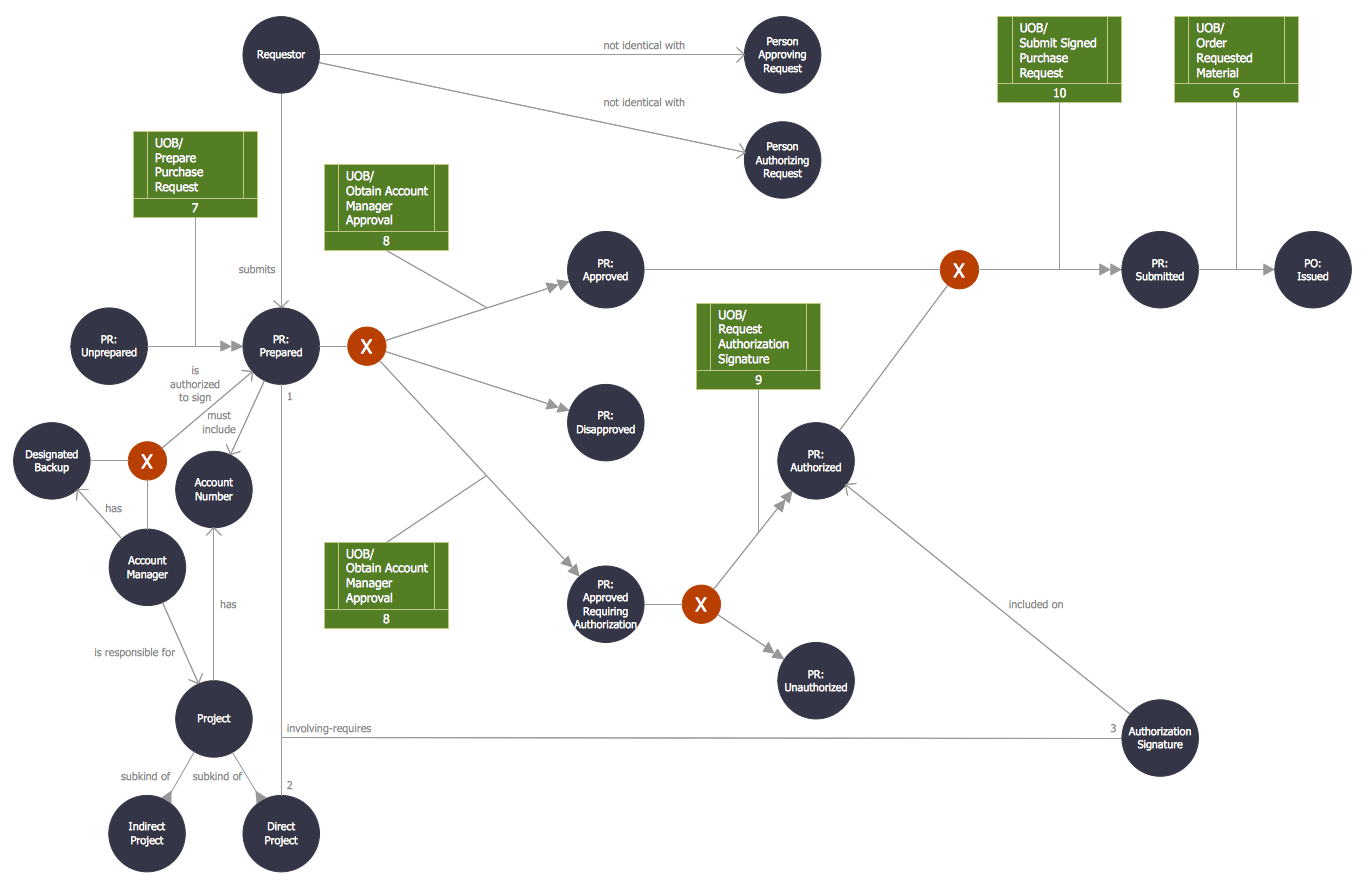Picture: Object-Oriented Design
Related Solution:

### SysML →

Systems Modeling Language (SysML) is a general-purpose modeling language for systems engineering applications. ConceptDraw DIAGRAM diagramming and vector drawing software was extended with SysML Solution from the Software Development Area of ConceptDraw Solution Park specially to help systems engineers design various model systems with SysML.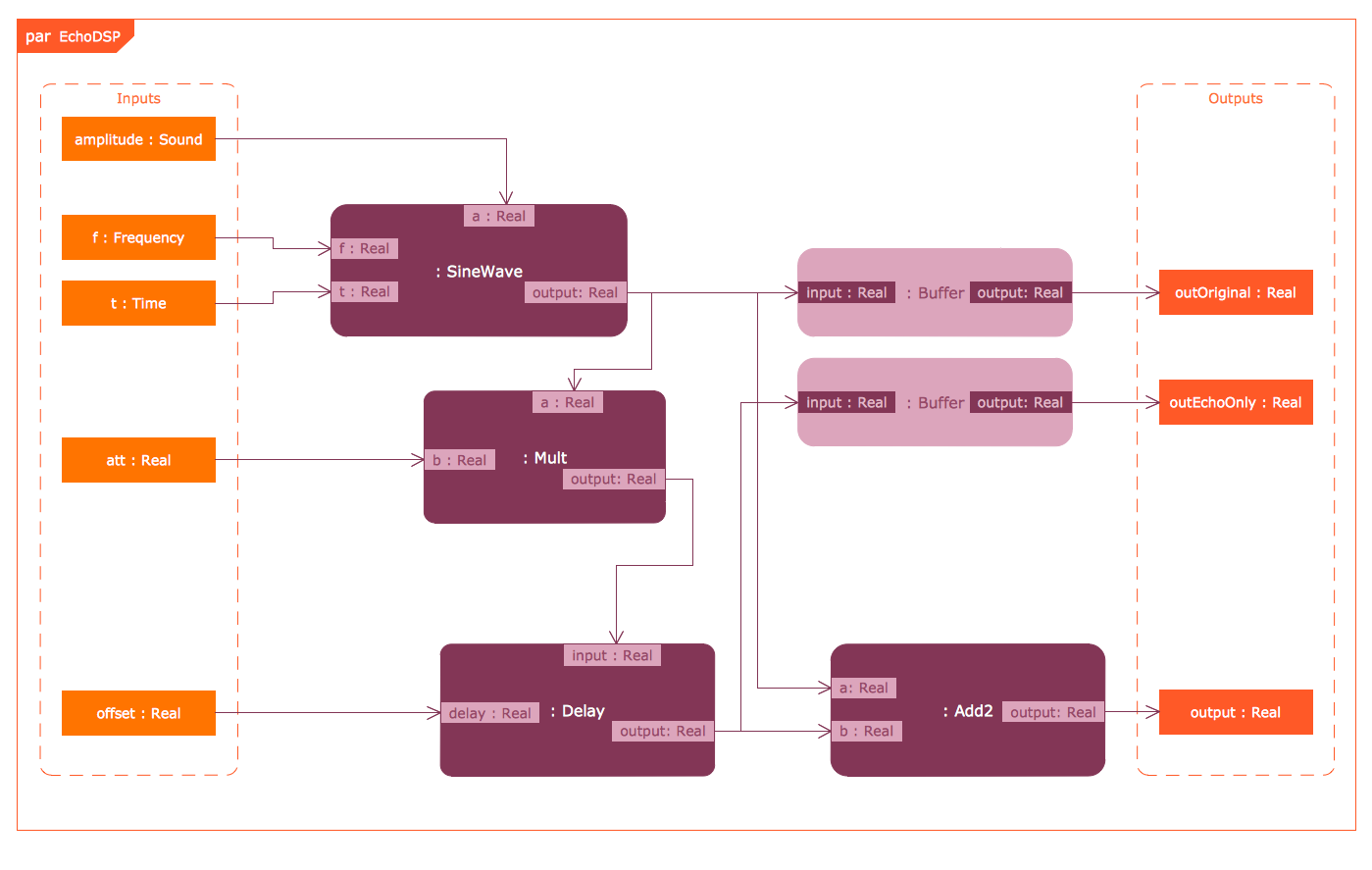Picture: SysML
Related Solution:
ConceptDraw
DIAGRAM 14